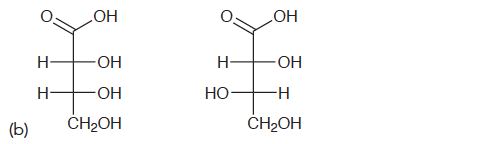Problem: For each of the following pairs of compounds, determine  the relationship between the two compounds:

FREE Expert Solution
88% (378 ratings)
Problem Details

For each of the following pairs of compounds, determine  the relationship between the two compounds:What scientific concept do you need to know in order to solve this problem?

Our tutors have indicated that to solve this problem you will need to apply the What is the Relationship Between Isomers? concept. You can view video lessons to learn What is the Relationship Between Isomers? Or if you need more What is the Relationship Between Isomers? practice, you can also practice What is the Relationship Between Isomers? practice problems .

What is the difficulty of this problem?

Our tutors rated the difficulty of For each of the following pairs of compounds, determine  the... as medium difficulty.

How long does this problem take to solve?

Our expert Organic tutor, Jonathan took less than a minute to solve this problem. You can follow their steps in the video explanation above.

What textbook is this problem found in?

Our data indicates that this problem or a close variation was asked in . You can also practice practice problems .